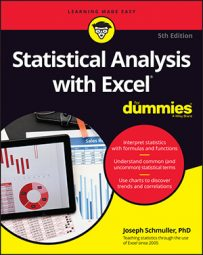##### Statistical Analysis with Excel For DummiesAmong the many tools that Excel provides for statistical analysis are some related to variation. Let’s take a quick look at these variation-related worksheet functions.

## DEVSQ

`DEVSQ` calculates the sum of the squared deviations from the mean (without dividing by N or by N-1). For these numbers

50, 47, 52, 46, and 45

that's 34.

Although it’s rare to calculate just the sum of squared deviations, you may find a need to apply this function.

## Average deviation

One more Excel function deals with deviations in a way other than squaring them.

The variance and standard deviation deal with negative deviations by squaring all the deviations before averaging them. How about if you just ignore the minus signs? This is called taking the absolute value of each deviation. (That's the way mathematicians say "How about if we just ignore the minus signs?")

If you do that for the heights

50, 47, 52, 46, and 45

you can put the absolute values of the deviations into a table like this one.

 Height Height-Mean |Deviation| 50 50-48 2 47 47-48 1 52 52-48 4 46 46-48 2 45 45-48 3

Notice the vertical lines around Deviation in the heading for the third column. Vertical lines around a number symbolize its absolute value. That is, the vertical lines are the mathematical symbol for "How about if we just ignore the minus signs?"

The average of the numbers in the third column is 2.4. This average is called the average absolute deviation, and it's a quick and easy way to characterize the spread of measurements around their mean. It’s in the same units as the original measurements. So if the heights are in inches, the absolute average deviation is in inches, too.

Like variance and standard deviation, a large average absolute deviation signifies a lot of spread. A small average absolute deviation signifies little spread.

This statistic is less complicated than variance or standard deviation, but is rarely used. Why? Statisticians can't use it as the foundation for additional statistics you meet later. Variance and standard deviation serve that purpose.

## AVEDEV

Excel's `AVEDEV` worksheet function calculates the average absolute deviation of a group of numbers. The image below shows the `AVEDEV` dialog box, which presents the average absolute deviation for the cells in the indicated range.